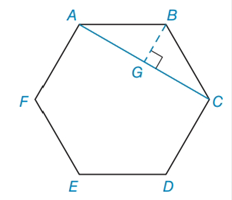Chapter 8.3, Problem 32E### Elementary Geometry for College St...

6th Edition
Daniel C. Alexander + 1 other
ISBN: 9781285195698

#### Solutions

Chapter
Section### Elementary Geometry for College St...

6th Edition
Daniel C. Alexander + 1 other
ISBN: 9781285195698
Textbook Problem
1 views

# Given regular hexagon A B C D E F with each side of the length 6 and the diagonal A C ¯ , find the area of pentagon A C D E F .To determine

To find:

The area of pentagon ACDEF.

Explanation

Calculation:

Given,

and the length of each side is 6

Calculation:

Consider the right angle triangle BGC.

The triangle  BGC is the form of 306090. Therefore by the properties of 306090 the hypotenuse is equal to twice the length of the shorter leg, which is the side across from the 30 angle. The longer leg, which is across from the 60 angle, is equal to multiplying the shorter leg by the 3.

i.e., BC=2BG, GC=3 ·BG

since BC=6,

2BG=6

BG=3

and

GC=3 ·BG

GC=33

From the figure AC=AG+GC

### Still sussing out bartleby?

Check out a sample textbook solution.

See a sample solution

#### The Solution to Your Study Problems

Bartleby provides explanations to thousands of textbook problems written by our experts, many with advanced degrees!

Get Started

#### Evaluate the definite integrals in Problems 1-32.

Mathematical Applications for the Management, Life, and Social Sciences

#### Find the limit. limt0tan6tsin2t

Single Variable Calculus: Early Transcendentals, Volume I

#### What is the lowest score in the following distribution?

Essentials of Statistics for The Behavioral Sciences (MindTap Course List)

#### In Exercises 25-30, find the rules for the composite functions f g and g f. 30. f(x)=x+1;g(x)=1x1

Applied Calculus for the Managerial, Life, and Social Sciences: A Brief Approach

#### n=12(14)n converges to: a) 94 b) 2 c) 23 d) the series diverges

Study Guide for Stewart's Single Variable Calculus: Early Transcendentals, 8th